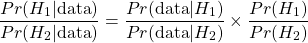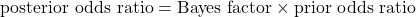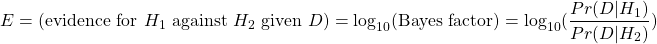## bookmark_borderQuantifying Evidence (2): Evidence Is Limited By How Much a Study Can Be Trusted

In part 1, we defined evidence and showed that evidence across independent studies can be aggregated by addition; if Alice’s results provide 2 units of evidence and Bob’s results provide 3 units of evidence then we have a total of 5 units of evidence. The problem with this is that it doesn’t account for our intuition that single experiments cannot be trusted too much until they are replicated. 10 congruent studies each reporting 2 units of evidence should prevail over one conflicting study showing -20 units of evidence.

Let’s try to model this by assuming that every experiment has a chance of being flawed due to some mistake or systematic error. Each study can have its own probability of failure, in which case the results of that experiment should not be used at all. This is our first assumption: that any result is either completely valid or completely invalid. It is a simplification but a useful one.

We define trust (T) in a particular study as the logarithm of the odds ratio for the being valid versus being invalid. In formal terms:

Continue reading “Quantifying Evidence (2): Evidence Is Limited By How Much a Study Can Be Trusted”

## bookmark_borderQuantifying Evidence (1): What Are Units of Evidence?

I am going to introduce a statistical framework for quantifying evidence as a series of blog posts. My hope is that by doing it through this format, people will understand it, build on these ideas, and actually use it as a practical replacement for p-value testing. If you haven’t already seen my post on why standard statistical methods that use p-values are flawed, you can check it out through this link.

My proposal builds on Bayesian hypothesis testing. Bayesian hypothesis testing makes use of the Bayes factor, which is the likelihood ratio of observing some data D for two competing hypotheses H1 and H2. A Bayes factor larger than 1 counts as evidence in favor of hypothesis H1; a smaller than one Bayes factor counts as evidence in favor of H2.

In classical hypothesis testing, we typically set a threshold for the p-value (say, p<0.01) below which a hypothesis can be rejected. But in the Bayesian framework, no such threshold can be defined as hypothesis rejection/confirmation will depend on the prior probabilities. Prior probabilities (i.e., the probabilities assigned prior to seeing data) are subjective. One person may assign equal probabilities for H1 and H2. Another may think H1 is ten times more likely than H2. And neither can be said to be objectively correct. But the Bayesian method leaves this subjective part out of the equation, allowing anyone to multiply the Bayes factor into their own prior probability ratio to obtain a posterior probability ratio. Depending on how likely you think the hypotheses are, you may require more or less evidence in order to reject one in favor of the other.Let us define ‘evidence‘ as the logarithm of the Bayes factor. The logarithmic scale is much more convenient to work with, as we will quickly see.Evidence is a quantity that depends on a particular observation or outcome and relates two hypothesis to one another. It can be positive or negative. For example, one can say Alice’s experimental results provide 3 units of evidence in favor of hypothesis H1 against hypothesis H2, or equivalently, -3 units of evidence in favor of hypothesis H2 against hypothesis H1.Figure 1: In this example, two hypotheses are being tested against one another. H1 is the hypothesis that the opossum is genetically closer to humpback whales than salmon is. H2 is the hypothesis that salmon is closer to humpback whales than the opossum. The data D that is being used to compare these hypotheses can be some DNA sequencing data, for instance. (The data here isn’t real).
Continue reading “Quantifying Evidence (1): What Are Units of Evidence?”